Favorite Problems
Seymour Papert in his seminal work "Mindstorms: Children, Computers and Powerful Ideas" (1980) writes about a classic problem from the perspective of trying to get inside and understand the mathematics behind our intuition that seems to go awry. Here's the description of the problem in Papert's own words.

The String Bridge Above the Earth Problem
from Mindstorms, (page 146-150):
by Seymour Papert
Imagine a string around the circumference of  the earth. Someone makes a proposal to place the string on six-foot-high poles. Obviously this implies that the string will have to be longer. But how much longer? Before trying to figure it out, try a guess: Is it about one thousand miles longer, about a hundred, or about ten?  The challenge here is to intuit an approximate answer rather than calculate an exact one.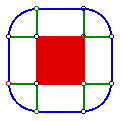The figure shows a string around the earth supported by poles of greatly exaggerated height. Call the radius of the earth R and the height of the poles H. The problem is to estimate the difference in length between the outer circumference and the true circumference. This is easy to calculate from the formula: C = 2pi*R. So the difference must be 2pi(R+h) - 2piR which is simply 2pi*h. But the challenge here is to "intuit" an approximate answer rather than calculate an exact one.

Most people who have the discipline to think before calculating - a discipline that forms part of the know-how of debugging one's intuitions - experience a compelling intuitive sense that "a lot of extra string is needed." For some the source of this conviction seems to lie in the idea that something is being added all around the twenty-four thousand miles (or so) off the earth's circumference. Others attach it to more abstract considerations of proportionality. But whatever the source, of the conviction may be it is "incorrect."  In anticipating the result of the the formal calculation which turns out to be a little less than 40 feet. The conflict between intuition and calculation is so powerful that the problem has become widely known as a teaser. And the conclusion that is often drawn from this conflict is that intuitions are not to be trusted. Instead of drawing this conclusion, we shall attempt to engage the reader in a dialog in order to identify what needs to be done to alter this intuition.  As a first step we follow the principle of seeking out a similar problem that might be more tractable. And a good general rule for simplification is to look for a linear version. Thus we pose the same problem on the assumption of a "square earth."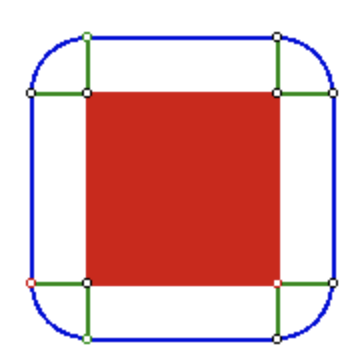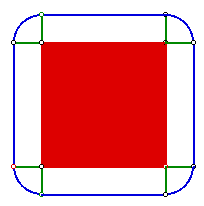The string on poles is assumed to be at distance H from the square. Increasing the size of the square does not change the quarter-circle pie slices. So the extra string needed to raise a string from the ground to height h is the same for a very small earth as for a very large one. The diagram gives us a geometric way to see that the same amount of extra string is needed here as in the case of the circle. This is itself quite startling. But more startling is the fact that we can see so directly that the size fo the square makes no difference to how much to how much extra string is needed. We could have calculated this fact by formula. But doing so would have left us in the same difficulty. By seeing it geometrically we can bring this case into line with our intuitive principle: Extra string is needed only where the earth curves. Obviously no extra string is needed to raise a straight line from the ground to a six-foot height.

Unfortunately this way of understanding the square case might seem to undermine our understanding of the circular case, We have completely understood the square but did so by seeing it as being very much different from the circle.

But there is another powerful idea that can come to the rescue. This is the idea of intermediate cases. When there is conflict between two cases, look for intermediates.

But what is intermediate between a square and a circle? Anyone who has studied calculus or Turtle geometry will have an immediate answer: polygons with more and more sides. So we look at figure (below) which shows strings around a series of polygonal earths. We see that the extra string needed remains the same in all these cases and, remarkably, we see something that might erode the argument that the circle adds something all around. The 1000-gon adds something at many more places. But it adds less, in fact one two hundred fiftieth at each of them.

Now will your mind take the jump? I have said nothing so far to come to this crucial step by rigorous argument. Nor shall I. But at this point some people begin to waver, and I conjecture that whether they do or not depends on how firm a commitment they have made to the idea of polygonal approximations to a circle. For those who have made to the idea of polygonal approximations to a circle. For those who have made the polygonal representation their own, the equivalence of polygon and circle is so immediate that intuition is carried along with it. People who do not yet "own" the equivalence between polygonal representation and circle can work at becoming better acquainted with it, for example, by using it to think through other problems.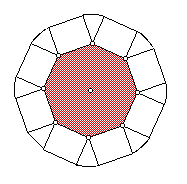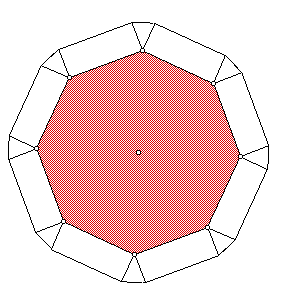In the octagon, too the extra string is all in the pie slices at the corners. If you put them together they form a circle of radius H. As in the case of the square, this circle is the same whether the octagon is small or big. What works for a the square (4-gon) and for the octagon (8-gon) works for the 100-gon and for the 1000-gon.

More on  this problem

Here's an interesting comment I found that was written by Gary Black:

 The important lesson is this: There is a particular way of understanding circles - a way that transcends the simple equation that relates circumference to diameter, but which is consistent with it - that allows a person to visualize the act of splicing in a length of circumference and permits them to predict accurately the new diameter. The person can successfully formulate an answer in the twinkling of an eye without lengthy calculation. If we didn't know the "trick" ourselves, and if we observed someone performing this experiment and witnessed them coming up with the correct answer over and over again, we might say the person possessed an "intuitive" understanding of circles, one that somehow allows the person to "see" or to "feel" the correct answer without performing an analysis. Moreover, by intuition we would not mean some weak, blind feeling, but rather a highly developed sense that is right every time.

A video solution to this bridge problem.

For more about Seymour Papert and Mindstorms see

http://www.stager.org/articles/LXeditorials/perspectivesonpapert.html
http://dailypapert.org
Edited 12.17.19 by Ihor Charischak